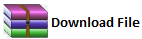Count Worksheets In Multiple Files

Consider a situation, where you are encountering with number of excel files on a daily basis & you want a quick mechanism to help you finding the count of worksheets present in each workbook. If you have a similar problem then you must not miss this article for it will help you a lot.

In this article, we will learn how to count worksheets in multiple files with VBA code.

Question: I need to macro that can read through a list of file names and return the number of worksheets that are present in each of the files (this is an audit mechanism to ensure that the correct number of worksheets are present in a series of files created via another process). The macro would need to establish the path of the folder where the files are located (all in the same folder), then locate the first file, identify the worksheet count, and repeat for the next file etc.

I thought I could do this with a formula by simply referencing the file names but I believe Excel does not have a straight forward formula for worksheet counts. Thanks!

Following is the snapshot of files saved in a folder with .xlsx extension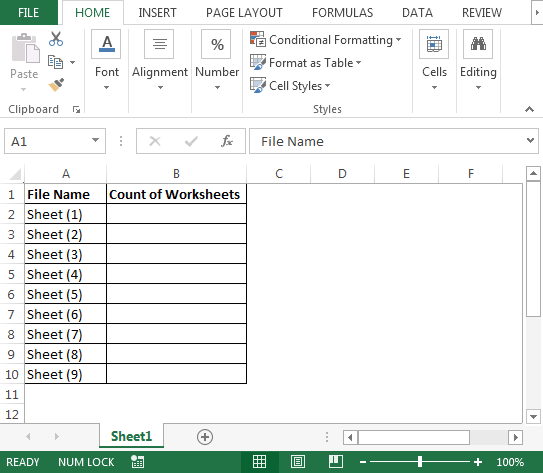Note: There are no password protected files.

To get the code, we need to follow the below steps to launch VB editor:

• Click on Developer tab
• From Code group, select Visual Basic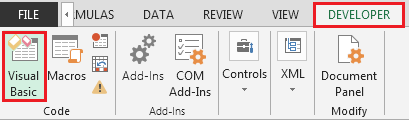• Copy the below code in the standard module

```Sub ListSheetCounts()
Dim Cell        As Range
Dim Conn        As Object
Dim Cat         As Object
Dim ConnStr     As String
Dim n           As Long
Dim Rng         As Range
Dim RngEnd      As Range
Dim WkbPath     As Variant
Dim Wks         As Worksheet

' Folder path where the workbooks are located.
WkbPath = "C:\Users\Test"

' Name of worksheet with the workbook list.
Set Wks = Worksheets("Sheet1")

' Starting cell of workbook list.
Set Rng = Wks.Range("A2")

' Get the range of cells in the workbook name list.
Set RngEnd = Wks.Cells(Rows.Count, Rng.Column).End(xlUp)
If RngEnd.Row >= Rng.Row Then Set Rng = Wks.Range(Rng, RngEnd)

' Create the needed ADO objects fro this macro.

' Add a final backslash the path if needed.
WkbPath = IIf(Right(WkbPath, 1) <> "\", WkbPath & "\", WkbPath)

' Step through each cell in the workbook list.
For Each Cell In Rng

' Get the worksheet count for the workbook.
ConnStr = "Provider=Microsoft.ACE.OLEDB.12.0;Data Source=" _
& WkbPath & Cell _
& ";Extended Properties=""Excel 12.0 Xml;HDR=YES;IMEX=1;"""
Conn.Open ConnStr

Set Cat.ActiveConnection = Conn

' Copy the count to the cell one column to right of the workbook name in the list.
Cell.Offset(n, 1) = Cat.Tables.Count

Conn.Close

Next Cell

' Clean up.
Set Cat = Nothing
Set Conn = Nothing
End Sub

```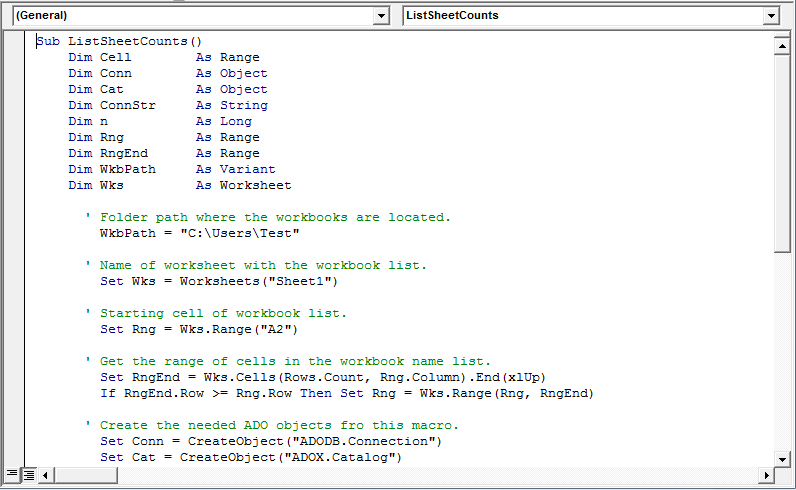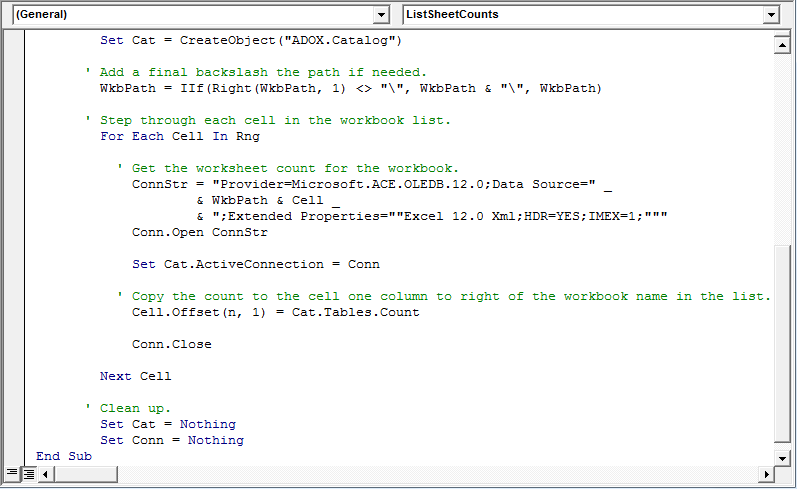• As we run the macro, we will get the number of worksheets. Refer below snapshot: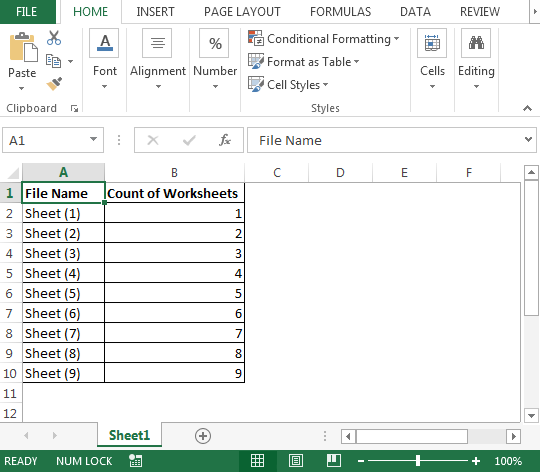Note: the above macro will work for .xlsx & .xls extension & not for .xlsm Macro enabled extension.

• All of the above files are .xlsx extension
• Let us add dummy excel sheet i.e. Sheet 10
• In case we have a file with same name having .xlsx & .xls extensions, then we need to mention the name of the file with their respective extensions as well in our test file (column A) so that macro can identify the file we are referring to & give us the correct result
• If we have not mentioned or have missed to mention the extension for the file with same name, then the macro will give us the count of .xlsx extension. Refer below snapshot: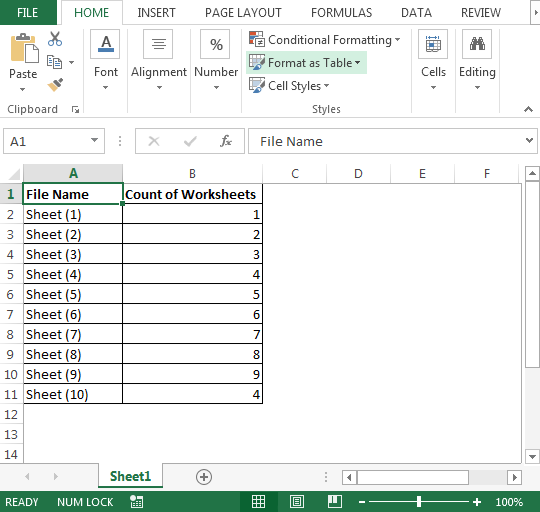• To get the number of sheets for Sheet 10 with .xlsx & .xls extensions, we need to mention the file name with their respective extensions

The snapshot of the final output is depicted below: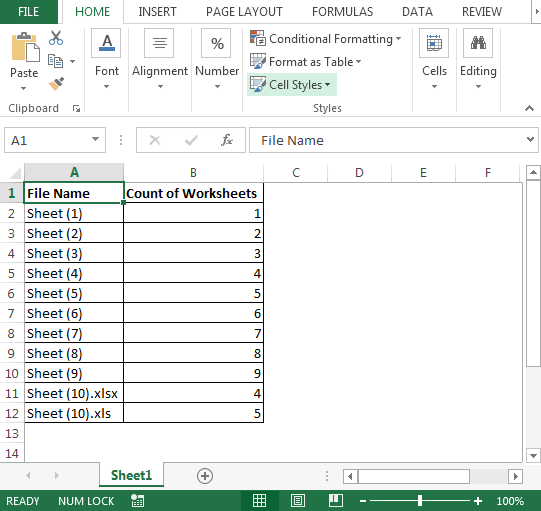Conclusion: Using above macro code we can count number of worksheets in multiple files & if require to get the custom result we can do little bit of modification in VBA code.